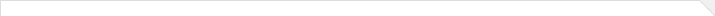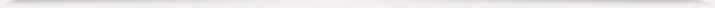报告题目： Boundary value problems for stationary compressible Navier--Stokes equations 报 告 人： Professor Pavel Plotnikov 报告人所在单位： Russian Academy of Sciences 报告日期： 2021-11-22 报告时间： 16:30 – 17:30 报告地点： ZOOM Meeting ID: 878 1712 1063 Passcode: 345958 报告摘要： This mini-course is devoted to the theory of boundary value problems for equations of viscous gas dynamics, named compressible Navier-Stokes equations. The mathematical theory of compressible Navier -Stokes equations is very interesting in its own right, but its principal significance lies in the central role Navier-Stokes equations now play in fluid dynamics. Most of these lectures concentrate on those aspects of the theory that have proven useful in applications.The main goal of the lecture course is to give an account of the basic principles of the theory of the Navier-Stokes equations for a compressible fluid. For simplicity, we restrict ourselves to the case of stationary boundary value problems. Along with the presentation of the classical results of the Lions-Feireisel theory, we will consider in detail the problem of the solvability of boundary value problems for the Navier-Stokes equations of the dynamics of real gases. In addition, we will touch upon little-studied problems of the dynamics of a viscous heat-conducting gas and inhomogeneous boundary value problems.Course structure and main topics:Physical background. The equations of the dynamics of a viscous gas. Basic principles of ThermodynamicsMathematical background. Elements of the theory of renormalized solutions of scalar hyperbolic equations. Lions-Meyer estimates for commutators of singular operators in Sobolev spaces. Concentrations of weak limits of functions from Sobolev spaces in critical cases. Integrability of functions from Sobolev spaces with respect to an arbitrary Borel measure. Monotonicity formulas for the Navier-Stokes equations.Solvability of the main boundary value problems for the compressible Navier-Stokes equations with a relaxation parameter. Energy estimation problem. Solvability of stationary boundary value problems for the Navier-Stokes equations with arbitrary adiabatic exponents.Stationary boundary value problems for the Fourier-Navier-Stokes equations for the dynamics of a viscous heat-conducting gas. The problem of the existence of solutions to inhomogeneous boundary value problems: existing results and their possible generalizations.11.22-12.01.pdf 本年度学院报告总序号： 318